## 2.21Scale similarity

Scale similarity is the notion that, for two systems that are geometrically similar, the ﬂow will follow the same path if the ratio of magnitude of forces acting on the ﬂuid is the same at diﬀerent points in the ﬂow.

Flows at diﬀerent scales can be compared using dimensionless variables. The momentum equation, Eq. (2.67 ), with advection, diﬀusion and gravity forces can be expressed in non-dimensionalised form by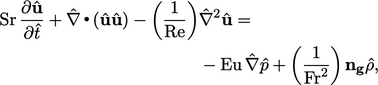(2.68)
with the dimensionless numbers21:
• Strouhal number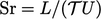— transient/steady inertia;
• Reynolds number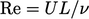— inertia/viscous force;
• Euler number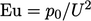— pressure force/inertia;
• (Froude number)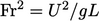— inertia/gravity force.

These dimensionless numbers include a characteristic length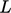, time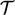, speed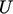and pressure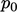. The(hat) notation indicates dimensionless length, time, etc., e.g.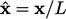and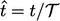and corresponding dimensionless operators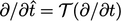and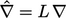.

Equation 2.68 assumes constant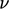and splits gravitational acceleration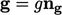into its magnitude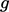and unit direction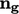; pressure, including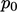, is in kinematic units (divided by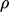).

The dimensionless numbers provide a comparison of the magnitudes of diﬀerent ﬂuid forces. For example,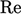represents the ratio of inertia force to viscous force and plays a pivotal role in turbulence modelling, introduced in Chapter 6 .

Scale similarity applies also to other transported properties. For example, the energy equation, Eq. (2.65 ), ignoring heat sources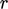, can be expressed in non-dimensional form as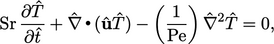(2.69)
which includes the following additional dimensionless number:22
• Péclet number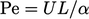— advection/diﬀusion of heat.

Again in Eq. (2.69 ), the(hat) notation is applied to temperature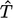to indicate a dimensionless temperature, although it notably does not appear in a dimensionless number.

In fact, with the exception of momentum (which uses),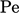represents more generally the rates of advection and diﬀusion, as a ratio, for any transported quantity (here, it is heat).

Further numbers23 deﬁne the ratios of diﬀusivities, e.g.:

• Prandtl number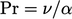— viscosity/thermal diﬀusivity;
• Schmidt number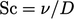— viscosity/mass diﬀusivity.

where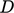is mass diﬀusivity (not discussed in this book).

Dimensionless numbers can be multiplied and divided with one another to form further dimensionless numbers. For example, the Péclet number for heat transfer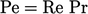.

21After Vincenc Strouhal (1850-1922), Osborne Reynolds (1842-1912), Leonhard Euler (1707-1783) and William Froude (1810-1879).
22After Jean Claude Eugène Péclet (1793-1857).
23After Ludwig Prandtl (1875-1953) and Ernst Heinrich Wilhelm Schmidt (1892-1975).

Notes on CFD: General Principles - 2.21 Scale similarity